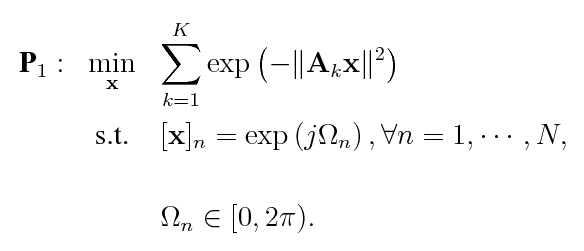# How to minimize sum-exp-quadratic problems

Hi, is somebody familiar with the following optimization problem?Any useful tips are appreciated.

I don’t know what “you” are doing with the j omega stuff (I presume complex angle representation), so I will just address the sum-exp-quadratic objective. It can be fairly straightforwardly entered into CVX.

``````cvx_begin
variablex(n)
minimize(exp(square_pos(norm(A1*x)))+exp(square_pos(norm(A2*x))))
cvx_end
``````

More generally, you can use a for loop with a 3 dimensional A array to build up the objective. If this isn’t sufficient help, you’ll have to clarify what you are trying to do.

Hi Mark,

Thank you for your help! But I think that you make a small mistake when you transform the objective function into CVX codes. There exists a negative symbol within the exponential function and you just omit it. Actually, I agree with you that without the negative symbol, the objective function is indeed convex. In this case, we can use Riemann manifold gradient to find the optimal solution. But unfortunately, my objective function seems non-convex, and thereby I cannot use CVX to solve it directly. I am sure whether I am right or not.

By the way, j = sqrt(-1) is the imaginary unit which you are correct.

Best regards,
Daniel

Whoops, that was a reado on my part.